### Adding a Flatten edges node

This node flattens the four edges of a terrain so that they are at zero altitude.

To add a Flatten edges node, right-click in the Graph Editor and select Create Node  > Terrain Transformation  > Flatten edges or press the keyboard shortcut N-T-F-E (Node Terrain Flatten Edges).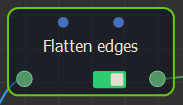Select the node to open its parameters: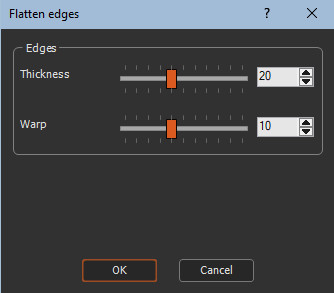### Editing a Flatten edges node

This node is generally used to easily add a terrain inside a larger terrain with a Full composition node.

To edit a Flatten edges node:

• Thickness: Defines the edge width relative to the total size of the terrain. For example, in the image below, the left image shows a thickness = 5 and the right image a thickness = 40.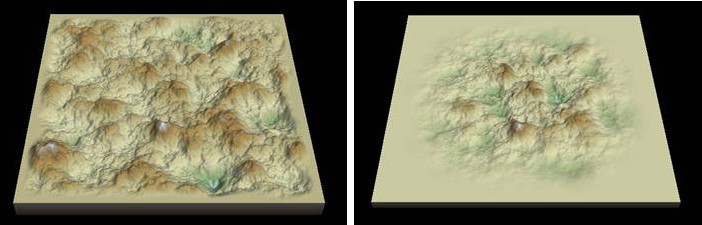• Warp: Distorts the edges to obtain a less regular result. For example, in the image below, the left image shows a warp = 0 and the right image a warp = 25.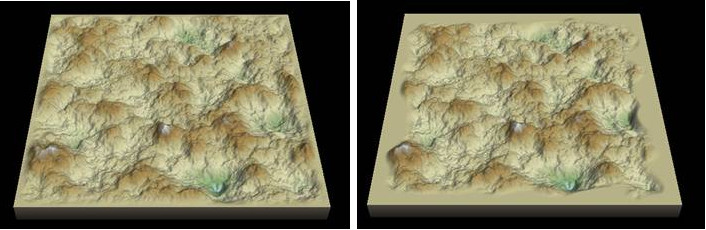Warning: If the average height of the terrain is much greater than zero or much less than zero, setting the edges to zero can lead to unsightly results. The example below shows the result obtained when the average height of the terrain  is much greater than zero.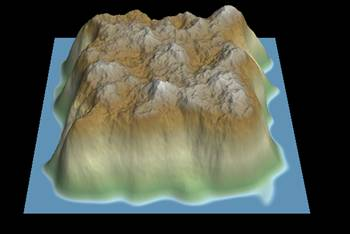Below is the result obtained when the average height of the terrain is much less than zero.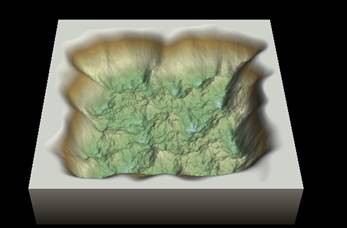In this case, it is advisable to adjust the height of the terrain using a Scale & offset node or a Min & max heights node.

### Parameters

Parameter Use
Thickness Defines the edge width relative to the total size of the terrain.
Warp Distorts the edges to obtain a less regular result.